1） 直截了当的实现

2） 高效的计算

3） 所需内存少

4） 梯度对角缩放的不变性

5） 适合解决含大规模数据和参数的优化问题

6） 使用于非稳态目标

7） 适用于解决包含很高噪声或稀疏梯度的问题

8） 超参数可以很直观地解释，并且基本上只需要极少量的调参

2）  均方根传播基于权重梯度最近量级的均值为每一个参数适应性的保留学习率。这意味着算法在非稳态和在线问题上有很优秀的性能

4）  移动均值的初始值和beta1、beta2值接近于1（推荐值），因此矩估计的偏差接近于0，该偏差通过首先计算带偏差的估计而后计算偏差修正后的估计而得到提升。如果对具体的实现细节和推导过程感兴趣，可以继续阅读第二部分和原论文

alpha同样也称为学习率或步长因子，它控制了权重的更新比率（如0.001）.较大的值（如0.3）在学习率更新前会更快的初始学习，而较小的值（如1E-5）会令训练收敛到更好的性能

beta1一阶矩估计的指数衰减率（如0.9）

beta2二阶矩估计的指数衰减率（如0.99）.该超参数在系数梯度（如在NLP或计算机视觉任务中）中应该设置接近1的数

epsilon该参数是非常小的数，其为了防止在实现中除以零（如1E-8）

TensorFlow：learning_rate=0.001, beta1=0.9, beta2=0.999, epsilon=1e-08.

Keras：lr=0.001, beta_1=0.9, beta_2=0.999, epsilon=1e-08, decay=0.0.

Blocks：learning_rate=0.002, beta1=0.9, beta2=0.999, epsilon=1e-08, decay_factor=1.

Lasagne：learning_rate=0.001, beta1=0.9, beta2=0.999, epsilon=1e-08

Caffe：learning_rate=0.001, beta1=0.9, beta2=0.999, epsilon=1e-08

MxNet：learning_rate=0.001, beta1=0.9, beta2=0.999, epsilon=1e-8

Torch：learning_rate=0.001, beta1=0.9, beta2=0.999, epsilon=1e-8

require：步长alpha：（建议默认为：0.001）

require：矩估计的指数衰减率，beta1、beta2在区间[0,1]内。（建议默认为：分别为0.9和0.999）

require:用于数值稳定的小常数epsilon（建议默认为：1E-8）

require：初始参数为

初始化一阶和二阶变量s=0，r=0

初始化时间步t=0

while 没有达到停止标准 do

从训练集中采包含m个样本的小批量，对应目标为。

计算梯度：

更新有偏一阶矩估计：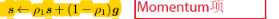更新有偏二阶矩估计：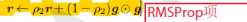修正一阶矩的偏差：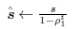修正二阶矩的偏差：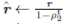计算更新：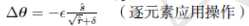应用更新：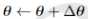end while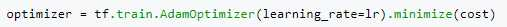(0)
(1)